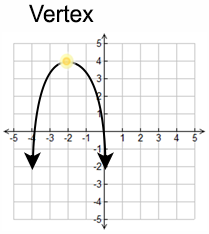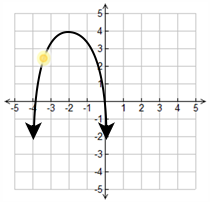Vertex (algebraic)

My Definition

Key Characteristics

The following are true of a vertex (algebraic):

• The vertex can be a minimum or maximum point.

• For parabolas opening up or down, the x-value of the vertex is equidistant from the x values of the x-intercepts.

• For parabolas opening up or down, the y-value of the vertex is equidistant from the y value of the focus and the closest point on the directrix.

• For parabolas opening up or down, the x-value of the vertex is same as the x-value of all the points on the axis of symmetry.

• The x-value can be found using the formula h =
-b2a
for a parabola with the equation y = ax2 + bx + c.

• The y-value can be found by using the x-value into the equation, y = ax2 + bx + c, and simplifying.

• Similar statements can be made for parabolas opening to the left and right.

ExampleNon-exampleTEKS: A(6)(B), A(7)(A)